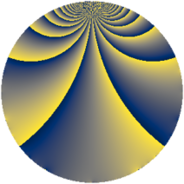# Properties

 Label 441.2.fLevel $441$ Weight $2$ Character orbit 441.f Rep. character $\chi_{441}(148,\cdot)$ Character field $\Q(\zeta_{3})$ Dimension $72$ Newform subspaces $8$ Sturm bound $112$ Trace bound $3$

# Related objects

## Defining parameters

 Level: $$N$$ $$=$$ $$441 = 3^{2} \cdot 7^{2}$$ Weight: $$k$$ $$=$$ $$2$$ Character orbit: $$[\chi]$$ $$=$$ 441.f (of order $$3$$ and degree $$2$$) Character conductor: $$\operatorname{cond}(\chi)$$ $$=$$ $$9$$ Character field: $$\Q(\zeta_{3})$$ Newform subspaces: $$8$$ Sturm bound: $$112$$ Trace bound: $$3$$ Distinguishing $$T_p$$: $$2$$, $$5$$

## Dimensions

The following table gives the dimensions of various subspaces of $$M_{2}(441, [\chi])$$.

Total New Old
Modular forms 128 92 36
Cusp forms 96 72 24
Eisenstein series 32 20 12

## Trace form

 $$72q + 2q^{2} + 4q^{3} - 30q^{4} - 2q^{5} + 8q^{6} - 24q^{8} + 8q^{9} + O(q^{10})$$ $$72q + 2q^{2} + 4q^{3} - 30q^{4} - 2q^{5} + 8q^{6} - 24q^{8} + 8q^{9} + 6q^{11} - 16q^{12} - 8q^{15} - 18q^{16} + 12q^{17} - 14q^{18} + 12q^{19} - 22q^{20} - 12q^{22} + 16q^{23} + 12q^{24} - 18q^{25} + 8q^{26} - 20q^{27} - 42q^{30} - 6q^{31} + 26q^{32} - 8q^{33} + 6q^{34} + 8q^{36} + 12q^{37} + 14q^{38} + 26q^{39} + 12q^{40} - 22q^{41} - 12q^{43} - 44q^{44} - 14q^{45} - 6q^{47} + 14q^{48} - 18q^{50} - 68q^{51} - 18q^{52} + 16q^{53} + 44q^{54} + 12q^{55} - 18q^{57} - 18q^{58} - 12q^{59} + 62q^{60} + 96q^{62} - 24q^{64} + 24q^{65} - 34q^{66} - 12q^{67} + 12q^{68} + 48q^{69} - 60q^{71} + 126q^{72} + 36q^{73} - 22q^{74} - 22q^{75} - 6q^{76} - 30q^{78} + 8q^{80} - 16q^{81} - 30q^{83} + 18q^{85} + 58q^{86} - 8q^{87} - 6q^{88} - 20q^{89} - 100q^{90} - 4q^{92} + 4q^{93} + 6q^{94} - 26q^{95} - 32q^{96} + 44q^{99} + O(q^{100})$$

## Decomposition of $$S_{2}^{\mathrm{new}}(441, [\chi])$$ into newform subspaces

Label Dim. $$A$$ Field CM Traces $q$-expansion
$$a_2$$ $$a_3$$ $$a_5$$ $$a_7$$
441.2.f.a $$2$$ $$3.521$$ $$\Q(\sqrt{-3})$$ None $$-1$$ $$-3$$ $$-1$$ $$0$$ $$q+(-1+\zeta_{6})q^{2}+(-2+\zeta_{6})q^{3}+\zeta_{6}q^{4}+\cdots$$
441.2.f.b $$2$$ $$3.521$$ $$\Q(\sqrt{-3})$$ None $$-1$$ $$3$$ $$1$$ $$0$$ $$q+(-1+\zeta_{6})q^{2}+(2-\zeta_{6})q^{3}+\zeta_{6}q^{4}+\cdots$$
441.2.f.c $$6$$ $$3.521$$ $$\Q(\zeta_{18})$$ None $$-3$$ $$0$$ $$3$$ $$0$$ $$q+(-1+\zeta_{18}+\zeta_{18}^{5})q^{2}+(\zeta_{18}^{3}-\zeta_{18}^{5})q^{3}+\cdots$$
441.2.f.d $$6$$ $$3.521$$ 6.0.309123.1 None $$1$$ $$4$$ $$-5$$ $$0$$ $$q+(-\beta _{1}-\beta _{2}-\beta _{3}+\beta _{5})q^{2}+(-\beta _{2}+\cdots)q^{3}+\cdots$$
441.2.f.e $$10$$ $$3.521$$ 10.0.$$\cdots$$.1 None $$2$$ $$-1$$ $$4$$ $$0$$ $$q+\beta _{1}q^{2}-\beta _{4}q^{3}+(-1-\beta _{2}+\beta _{4}+\cdots)q^{4}+\cdots$$
441.2.f.f $$10$$ $$3.521$$ 10.0.$$\cdots$$.1 None $$2$$ $$1$$ $$-4$$ $$0$$ $$q+\beta _{1}q^{2}+\beta _{4}q^{3}+(-1-\beta _{2}+\beta _{4}+\cdots)q^{4}+\cdots$$
441.2.f.g $$12$$ $$3.521$$ $$\mathbb{Q}[x]/(x^{12} - \cdots)$$ None $$-2$$ $$0$$ $$0$$ $$0$$ $$q+(-\beta _{1}+\beta _{6})q^{2}+\beta _{10}q^{3}+(-1-\beta _{2}+\cdots)q^{4}+\cdots$$
441.2.f.h $$24$$ $$3.521$$ None $$4$$ $$0$$ $$0$$ $$0$$

## Decomposition of $$S_{2}^{\mathrm{old}}(441, [\chi])$$ into lower level spaces

$$S_{2}^{\mathrm{old}}(441, [\chi]) \cong$$ $$S_{2}^{\mathrm{new}}(63, [\chi])$$$$^{\oplus 2}$$